# Problem: House

Write a program that takes a number n (2 ≤ n ≤ 100) and prints a house with size n x n, just as in the examples:

Input Output Input Output Input Output
2 **
||
3 -*-
***
|*|
4 -**-
****
|**|
|**|
Input Output Input Output
5 --*--
-***-
*****
|***|
|***|
8 ---**---
--****--
-******-
********
|******|
|******|
|******|
|******|

## Video: Draw a House

Watch this video lesson to learn how to print a house on the console using nested loops: https://youtu.be/ExjxRM0vXW4.

## Hints and Guidelines

We understand from the problem explanation that the house is with size of n x n. What we see from the example input and output is that:

• The house is divided into two parts: roof and base.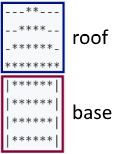• When n is an even number, the point of the house is "dull".
• When n is odd, the roof is one row larger than the base.

### The Roof

• It comprises of stars and dashes.
• In the top part there are one or two stars, depending on if n is even or odd (also related to the dashes).
• In the lowest part there are many stars and no dashes.
• With each lower row, the stars increase by 2 and the dashes decrease by 2.

### The Base

• The height is n rows.
• It is made out of stars and pipes.
• Each row comprises of 2 pipes – one in the beginning and one in the end of the row, and also stars between the pipes with string length of n - 2.

### Reading the Input Data

We read n from the console and we save it in a variable of int type.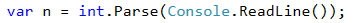It is very important to check if the input data is correct! In these tasks it is not a problem to directly convert the data from the console into int type, because it is said that we will be given valid integers. If you are making more complex programs it is a good practice to check the data. What will happen if instead of the character "А" the user inputs a number?

### Calculating Roof Length

In order to draw the roof, we write down how many stars we start with in a variable called stars:

• If n is an even number, there will be 2 stars.
• If it is odd, there will be 1.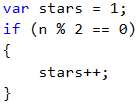Calculate the length of the roof. It equals half of n. Write the result in the variable roofLength.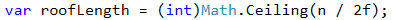It is important to note that when n is an odd number, the length of the roof is one row more than that of the base. In C# when you divide two numbers with a remainder, the result will be the number without remainder.

Example:

int result = 3 / 2; // result 1


If we want to round up, we need to use the method Math.Ceiling(…): int result = (int)Math.Ceiling(3 / 2f); In this example the division isn't between two integers. "f" after a number shows that this number is of float type (a floating-point number). The result of 3 / 2f is 1.5f. Math.Ceiling(…) rounds the division up. In this case 1.5f will become 2. (int) is used so that we can transfer the type back to int.

### Printing the Roof

After we have calculated the length of the roof, we make a loop from 0 to roofLength. On each iteration we will:

• Calculate the number of dashes we need to draw. The number will be equal to (n - stars) / 2. We store it in a variable padding.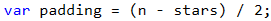• We print on the console: "dashes" (padding / 2 times) + "stars" (stars times) + "dashes" (padding / 2 times).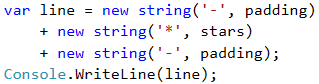• Before the iteration is over, we add 2 to stars (the number of the stars).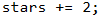It is not a good idea to add many character strings as it is shown above, because this leads to performance issues. Learn more at: https://en.wikipedia.org/wiki/String_(computer_science)#String_buffers

### Printing the Base

After we have finished with the roof, it is time for the base. It is easier to print:

• We start with a loop from 0 to n (not inclusive).
• We print on the console: | + * (n - 2 times) + |.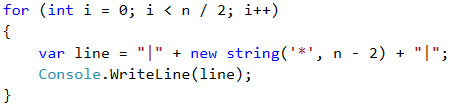If you have written everything as it is here, the problem should be solved.

## Testing in the Judge System

Test your solution here: https://judge.softuni.org/Contests/Practice/Index/512#8.